Precalculus : Use Product/Sum Identities to Express a Product as a Sum or Difference

Example Questions

Example Question #36 : Trigonometric Identities

Which of the following expressions best represent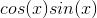?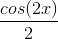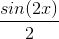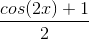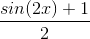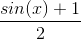Explanation:

Write the trigonometric product and sum identity for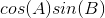.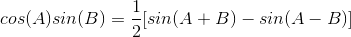For, replace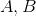withand simplify the expression.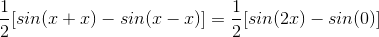Example Question #37 : Trigonometric Identities

Find the exact answer of: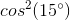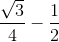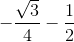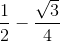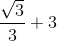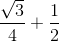Explanation:

The product and sum formula can be used to solve this question.

Write the formula for cosine identity.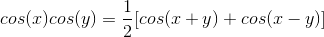Split up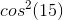into two separate cosine expressions.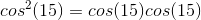Substitute the 2 known angles into the formula and simplify.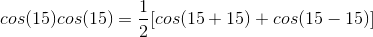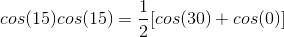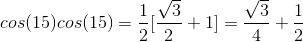Example Question #38 : Trigonometric Identities

Simplify the following. Leave your answer in terms of a trigonometric function.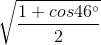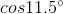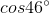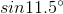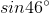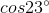Explanation:

This is a simple exercise to recognize the half angle formula for cosine.

The half angle formula for cosine is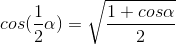.

In the expression given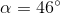With this in mind we can rewrite the expression as the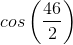, or, after dividing by two,Example Question #39 : Trigonometric Identities

Solve the following over the domainto.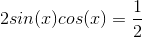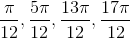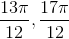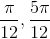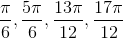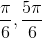Explanation:

Here we can rewrite the left side of the equation as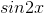because of the double angle formula for sin, which is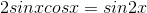.

Now our equation is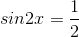,

and in order to get solve forwe take the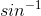of both sides. Just divide by two from there to findThe only thing to keep in mind here is that the period of the function is half of what it normally is, which is why we have to solve forand then addto each answer.Example Question #1 : Use Product/Sum Identities To Express A Product As A Sum Or Difference

Solve over the domainto.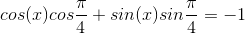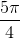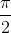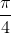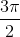Explanation:

We can rewrite the left side of the equation using the angle difference formula for cosine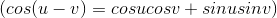as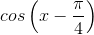.

From here we just take the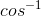of both sides and then addto get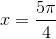.

Example Question #41 : Trigonometric Identities

Which expression is equivalent to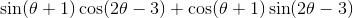?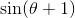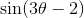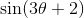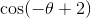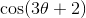Explanation:

The relevant trigonometric identity is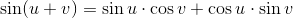In this case, "u" is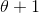and "v" is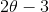.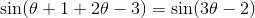.